# Recursion and Induction Themes Recursion Recurrence Relations Recursive

• Slides: 38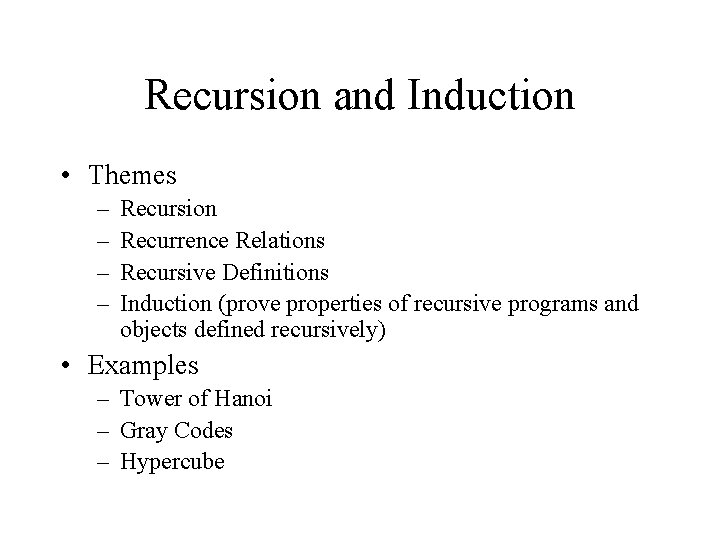Recursion and Induction • Themes – – Recursion Recurrence Relations Recursive Definitions Induction (prove properties of recursive programs and objects defined recursively) • Examples – Tower of Hanoi – Gray Codes – HypercubeTower of Hanoi • There are three towers • 64 gold disks, with decreasing sizes, placed on the first tower • You need to move all of the disks from the first tower to the last tower • Larger disks can not be placed on top of smaller disks • The third tower can be used to temporarily hold disks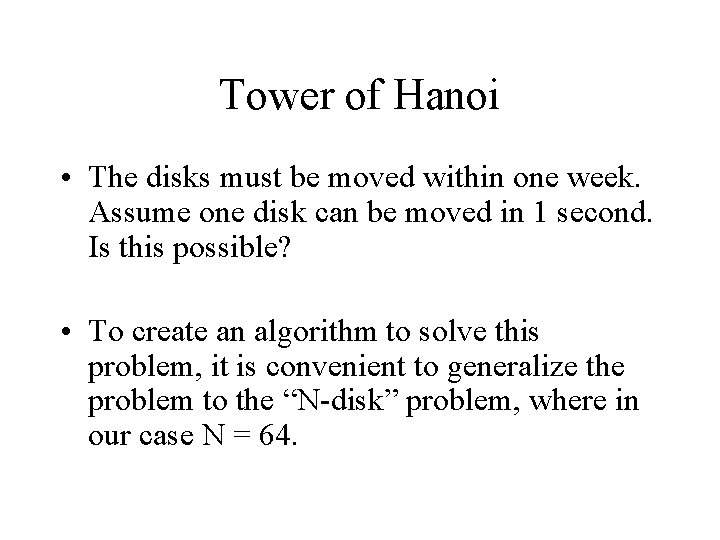Tower of Hanoi • The disks must be moved within one week. Assume one disk can be moved in 1 second. Is this possible? • To create an algorithm to solve this problem, it is convenient to generalize the problem to the “N-disk” problem, where in our case N = 64.Recursive SolutionRecursive SolutionRecursive Solution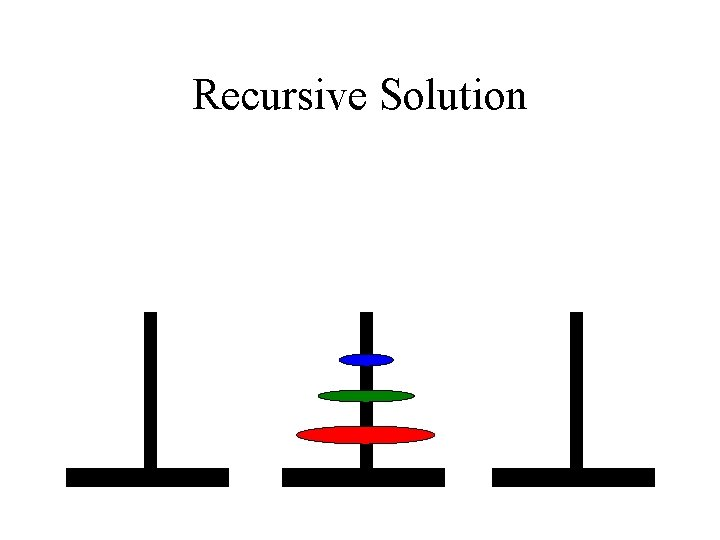Recursive SolutionTower of Hanoi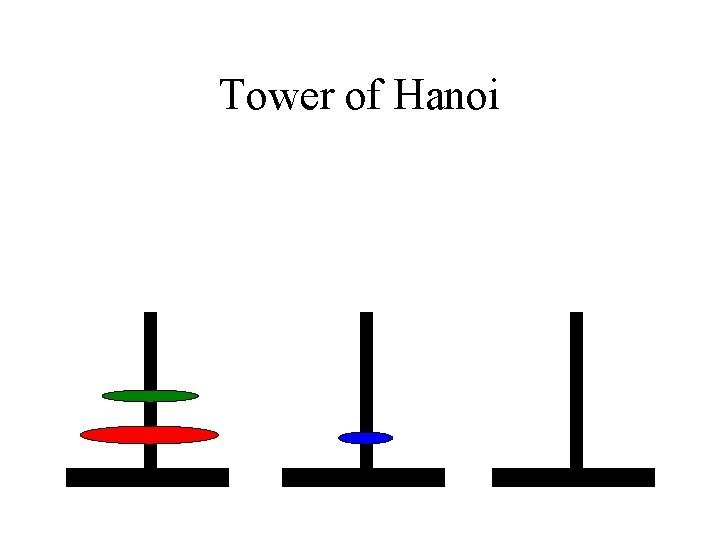Tower of HanoiTower of Hanoi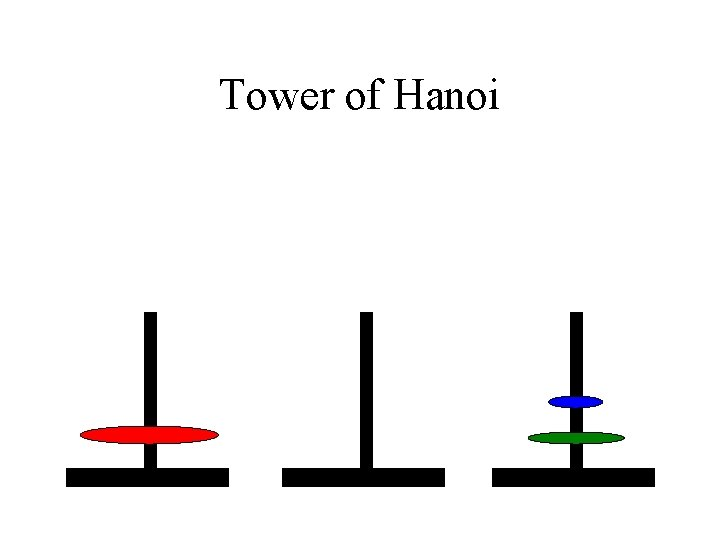Tower of HanoiTower of Hanoi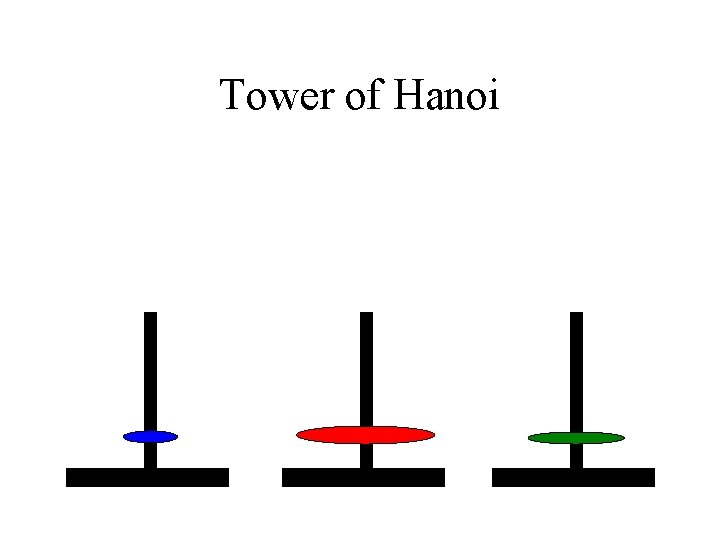Tower of Hanoi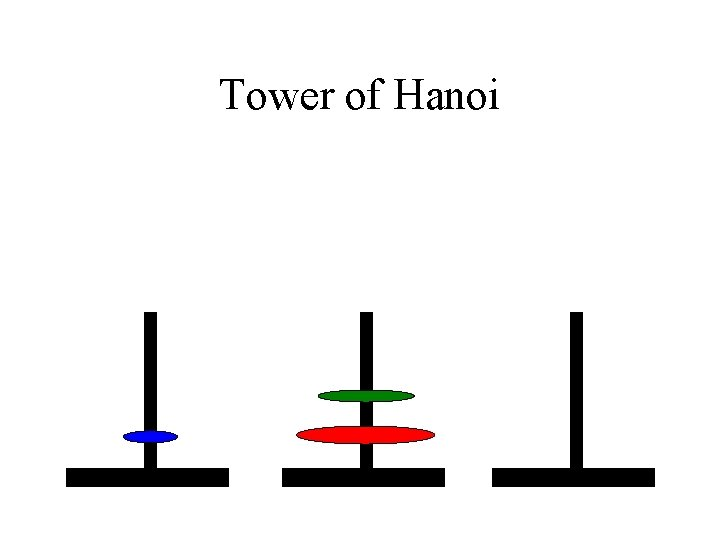Tower of Hanoi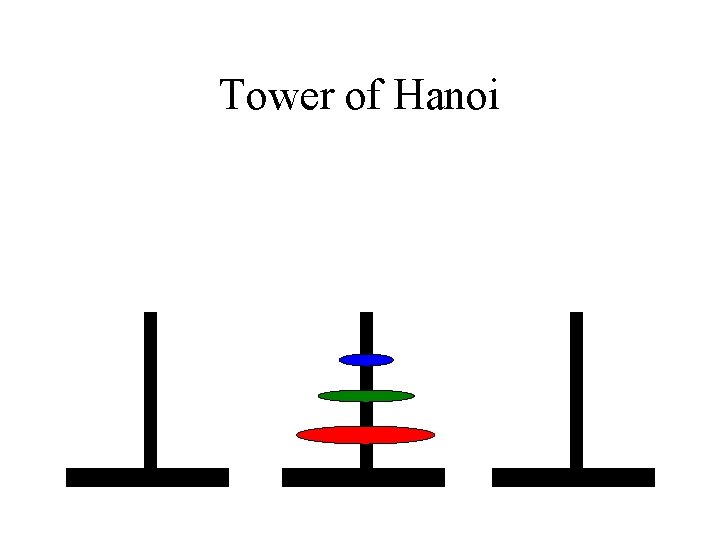Tower of Hanoi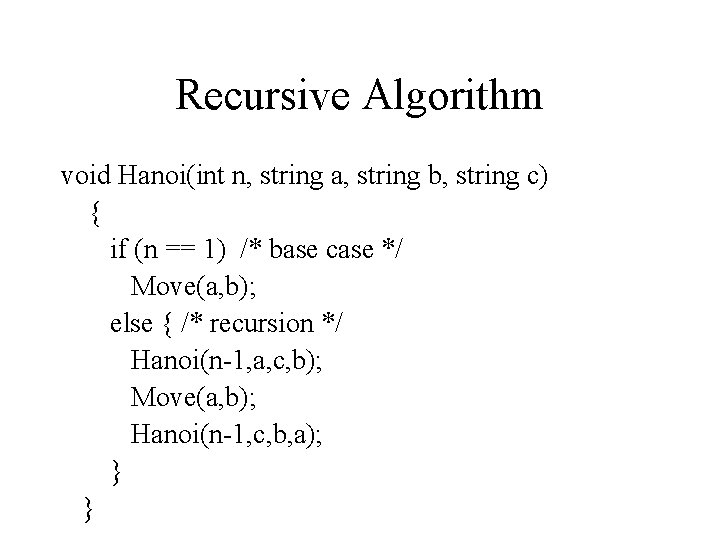Recursive Algorithm void Hanoi(int n, string a, string b, string c) { if (n == 1) /* base case */ Move(a, b); else { /* recursion */ Hanoi(n-1, a, c, b); Move(a, b); Hanoi(n-1, c, b, a); } }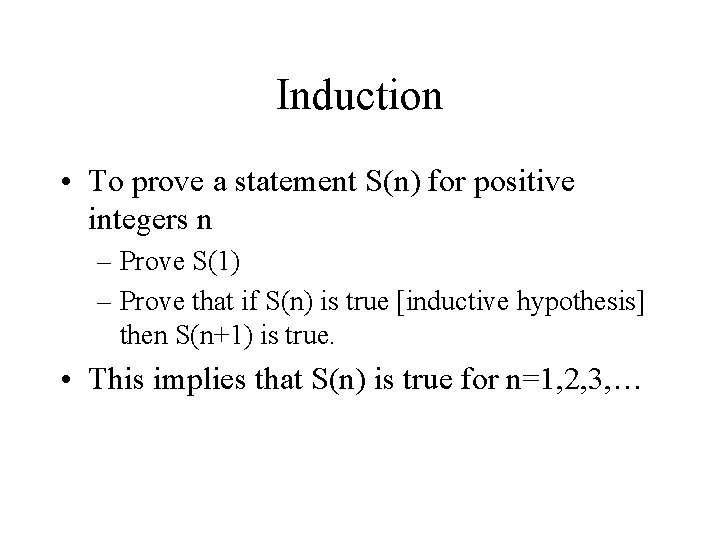Induction • To prove a statement S(n) for positive integers n – Prove S(1) – Prove that if S(n) is true [inductive hypothesis] then S(n+1) is true. • This implies that S(n) is true for n=1, 2, 3, …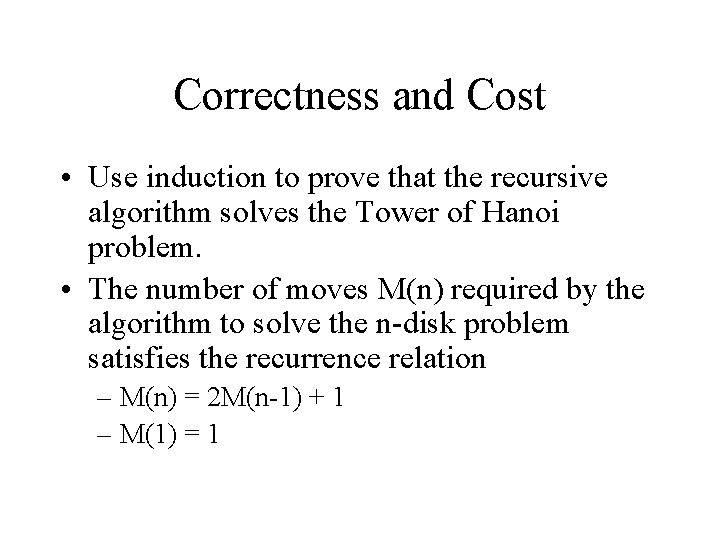Correctness and Cost • Use induction to prove that the recursive algorithm solves the Tower of Hanoi problem. • The number of moves M(n) required by the algorithm to solve the n-disk problem satisfies the recurrence relation – M(n) = 2 M(n-1) + 1 – M(1) = 1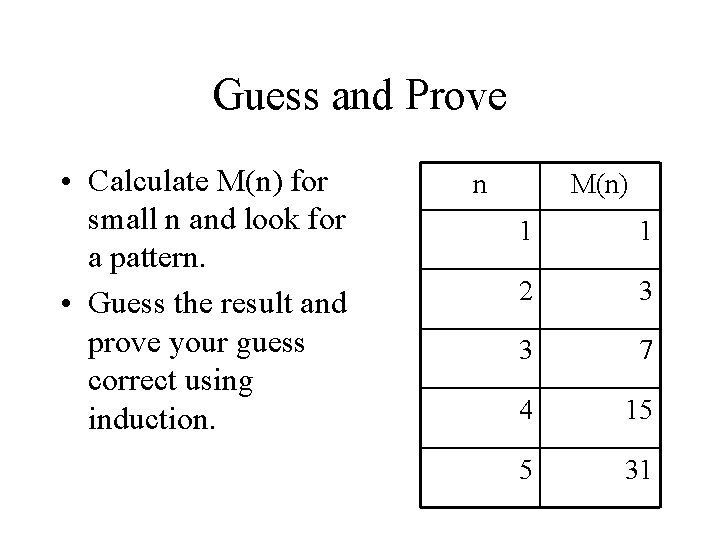Guess and Prove • Calculate M(n) for small n and look for a pattern. • Guess the result and prove your guess correct using induction. n M(n) 1 1 2 3 3 7 4 15 5 31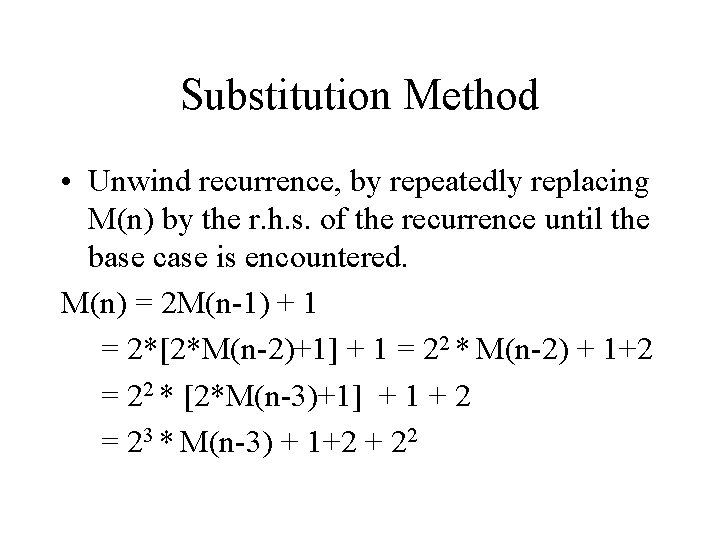Substitution Method • Unwind recurrence, by repeatedly replacing M(n) by the r. h. s. of the recurrence until the base case is encountered. M(n) = 2 M(n-1) + 1 = 2*[2*M(n-2)+1] + 1 = 22 * M(n-2) + 1+2 = 22 * [2*M(n-3)+1] + 1 + 2 = 23 * M(n-3) + 1+2 + 22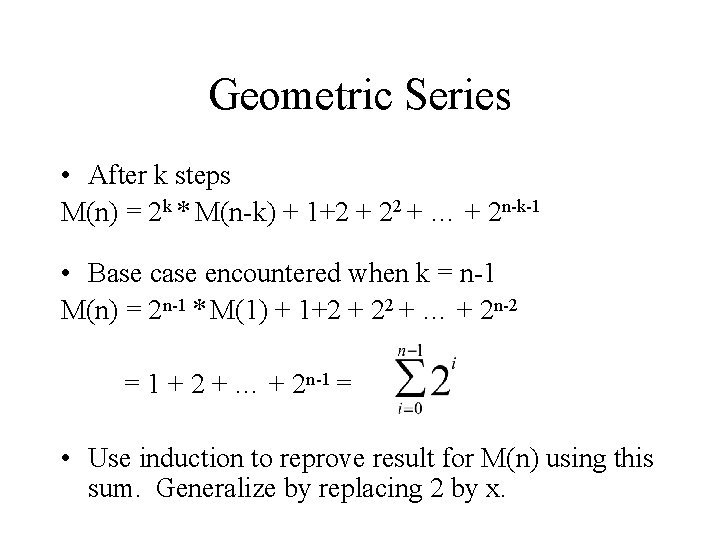Geometric Series • After k steps M(n) = 2 k * M(n-k) + 1+2 + 22 + … + 2 n-k-1 • Base case encountered when k = n-1 M(n) = 2 n-1 * M(1) + 1+2 + 22 + … + 2 n-2 = 1 + 2 + … + 2 n-1 = • Use induction to reprove result for M(n) using this sum. Generalize by replacing 2 by x.Gray Code • An n-bit Gray code is a 1 -1 onto mapping from [0. . 2 n-1] such that the binary representation of consecutive numbers differ by exactly one bit. • Invented by Frank Gray for a shaft encoder - a wheel with concentric strips and a conducting brush which can read the number of strips at a given angle. The idea is to encode 2 n different angles, each with a different number of strips, corresponding to the n-bit binary numbers.Shaft Encoder (Counting Order) 010 011 100 001 000 111 101 110 Consecutive angles can have an abrupt change in the number of strips (bits) leading to potential detection errors.Shaft Encoder (Gray Code) 011 010 110 001 000 111 101 Since a Gray code is used, consecutive angles have only one change in the number of strips (bits).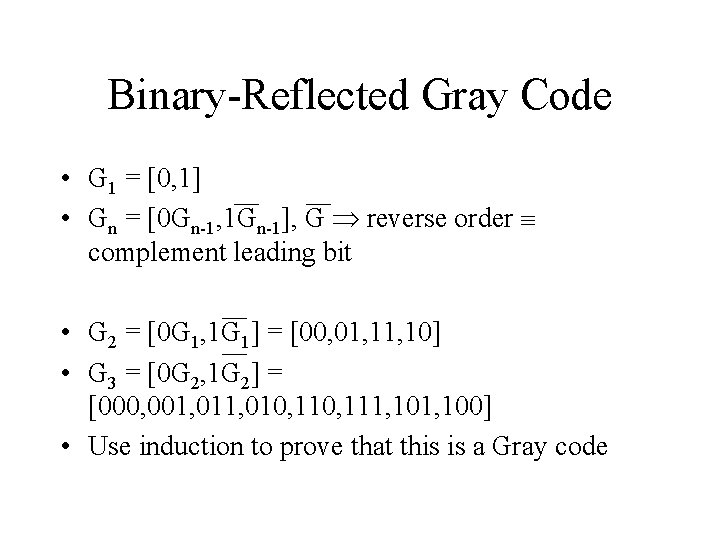Binary-Reflected Gray Code • G 1 = [0, 1] • Gn = [0 Gn-1, 1 Gn-1], G reverse order complement leading bit • G 2 = [0 G 1, 1 G 1] = [00, 01, 10] • G 3 = [0 G 2, 1 G 2] = [000, 001, 010, 111, 100] • Use induction to prove that this is a Gray codeIterative Formula • Let Gn(i) be a function from [0, …, 2 n-1] • Gn(i) = i ^ (i >> 1) [exclusive or of i and i/2] – G 2(0) = 0, G 2(1) = 1, G 2(2) = 3, G 2(3) = 2 • Use induction to prove that the sequence Gn(i), i=0, …, 2 n-1 is a binary-reflected Gray code.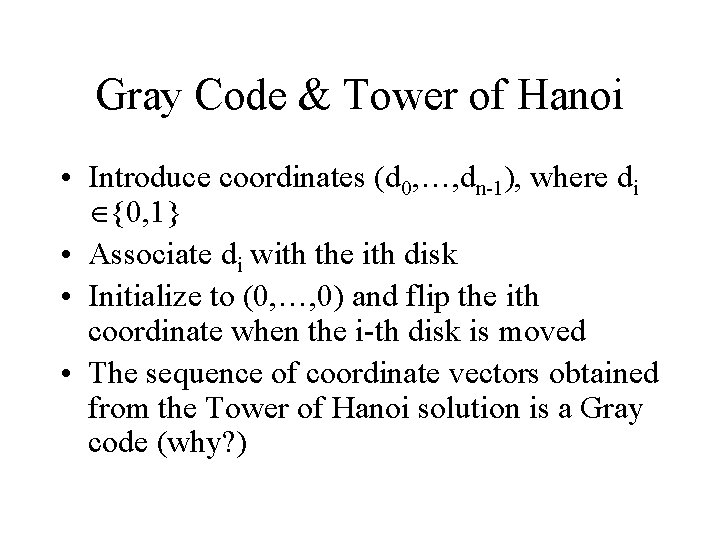Gray Code & Tower of Hanoi • Introduce coordinates (d 0, …, dn-1), where di {0, 1} • Associate di with the ith disk • Initialize to (0, …, 0) and flip the ith coordinate when the i-th disk is moved • The sequence of coordinate vectors obtained from the Tower of Hanoi solution is a Gray code (why? )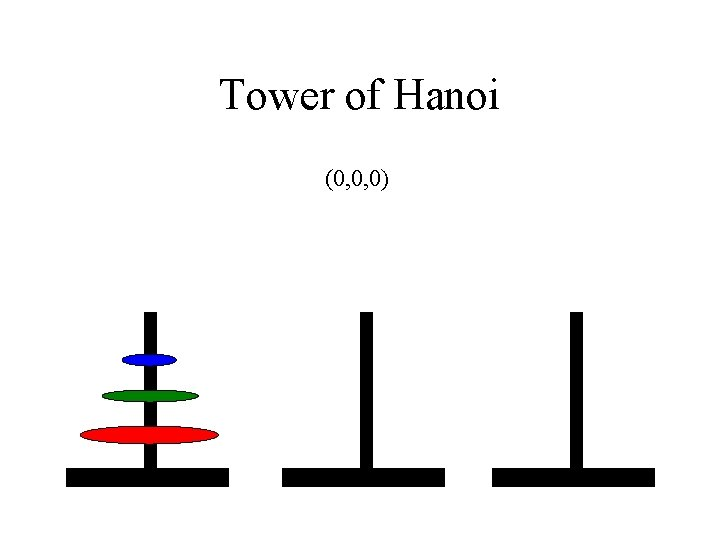Tower of Hanoi (0, 0, 0)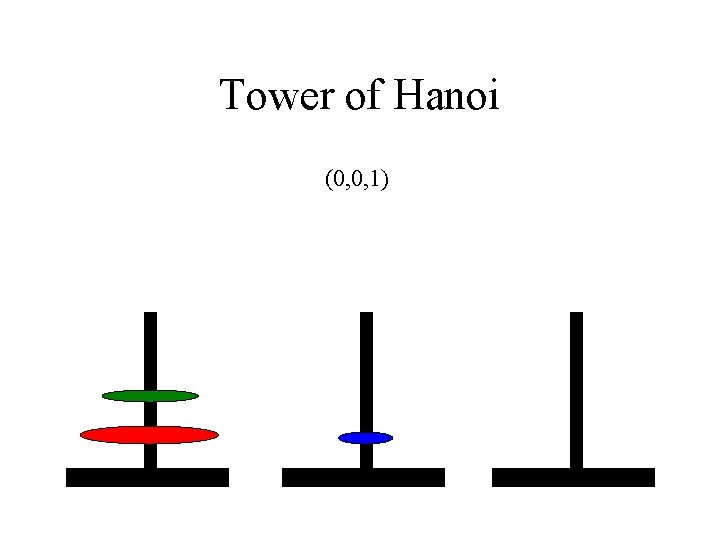Tower of Hanoi (0, 0, 1)Tower of Hanoi (0, 1, 1)Tower of Hanoi (0, 1, 0)Tower of Hanoi (1, 1, 0)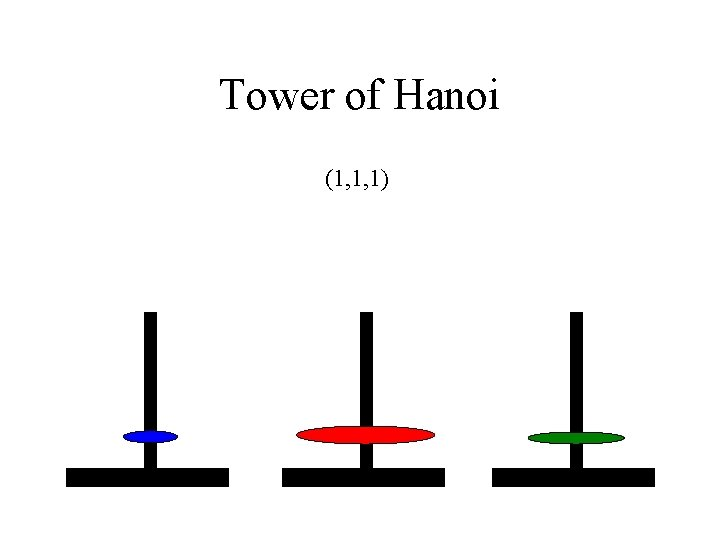Tower of Hanoi (1, 1, 1)Tower of Hanoi (1, 0, 1)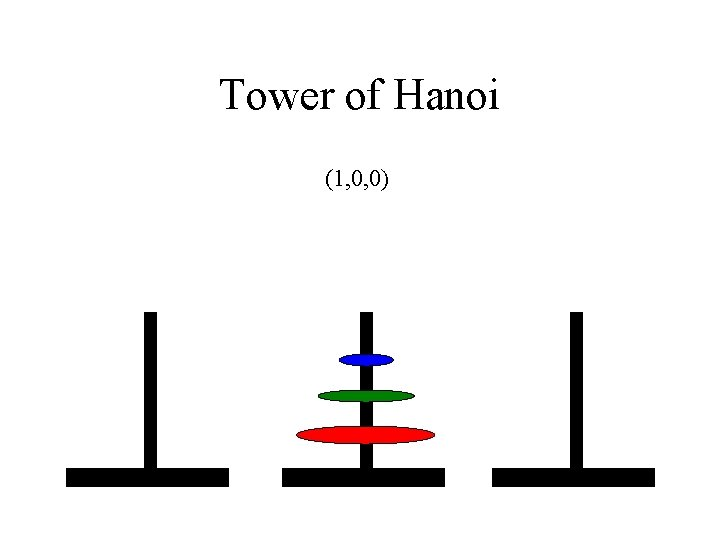Tower of Hanoi (1, 0, 0)Hypercube • Graph (recursively defined) • n-dimensional cube has 2 n nodes with each node connected to n vertices • Binary labels of adjacent nodes differ in one bit 110 111 10 0 11 1 00 011 010 100 01 000 101 001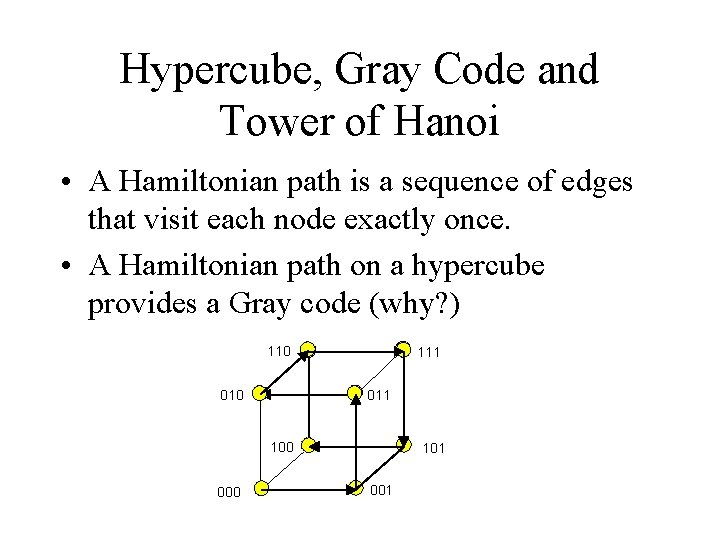Hypercube, Gray Code and Tower of Hanoi • A Hamiltonian path is a sequence of edges that visit each node exactly once. • A Hamiltonian path on a hypercube provides a Gray code (why? ) 110 111 010 100 000 101 001Hypercube and Gray Code 110 111 010 100 000 101 001